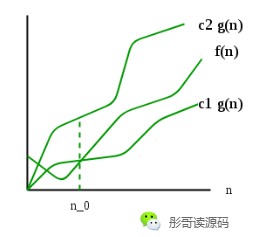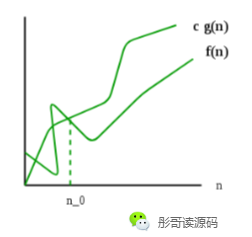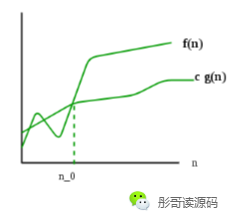### O、Θ、Ω、o、ω，别再傻傻分不清了！# 读音

• O，/əʊ/，大Oh
• o，/əʊ/，小oh
• Θ，/ˈθiːtə/，theta
• Ω，/oʊˈmeɡə/，大Omega
• ω，/oʊˈmeɡə/，小omega

# 数学解释

## Θ

Θ定义了一种精确的渐近行为（exact asymptotic behavior），怎么说呢？

``````对于f(n)，存在正数n0、c1、c2，使得当 n>=n0 时，始终存在 0 <= c1*g(n) <= f(n) <= c2*g(n)，则我们可以用 f(n)=Θ(g(n))表示。
``````Θ同时定义了上界和下界，f(n)位于上界和下界之间，且包含等号。

## O

O定义了算法的上界。

``````对于f(n)，存在正数n0、c，使得当 n>=n0 时，始终存在 0 <= f(n) <= c*g(n)，则我们可以用 f(n)=O(g(n))表示。
``````O只定义上界，只要f(n)不大于c*g(n)，就可以说 f(n)=O(g(n))。

1. 最坏的情况下，它的时间复杂度为Θ(n^2)；
2. 最好的情况下，它的时间复杂度为Θ(n)。

## o

o定义的也是算法的上界，不过它不包含等于，是一种不精确的上界，或者称作松上界（某些书籍翻译为非紧上界）。

``````对于f(n)，存在正数n0、c，使得当 n>n0 时，始终存在 0 <= f(n) < c*g(n)，则我们可以用 f(n)=o(g(n))表示。
``````o表示仅仅是大O去掉等于的情况，其他行为与大O一模一样。

## Ω

Ω定义了算法的下界，与O正好相反。

``````对于f(n)，存在正数n0、c，使得当 n>=n0 时，始终存在 0 <= c*g(n) <= f(n)，则我们可以用 f(n)=Ω(g(n))表示。
``````Ω只定义下界，只要f(n)不小于c*g(n)，就可以说 f(n)=Ω(g(n))。

## ω

ω同样定义的是下界，只不过不包含等于，是一种不精确的下界，或者称作松下界（某些书籍翻译为非紧下界）。

``````对于f(n)，存在正数n0、c，使得当 n>n0 时，始终存在 0 <= c*g(n) < f(n)，则我们可以用 f(n)=ω(g(n))表示。
``````ω表示仅仅是大Ω去掉等于的情况，其他行为与大Ω一模一样。

Θ 精确的渐近行为 相当于“=”
O 上界 相当于“<=”
o 松上界 相当于“<”
Ω 下界 相当于“>=”
ω 松下界 相当于“>”

# 后记